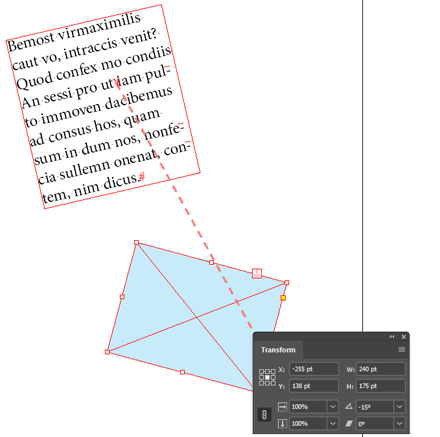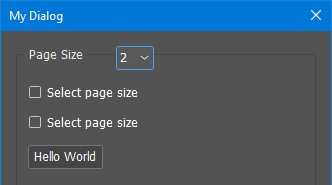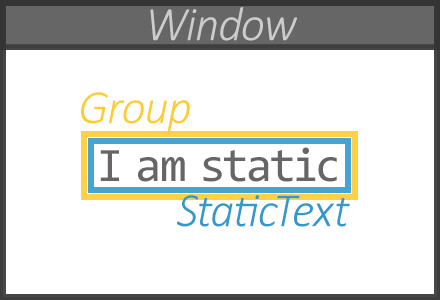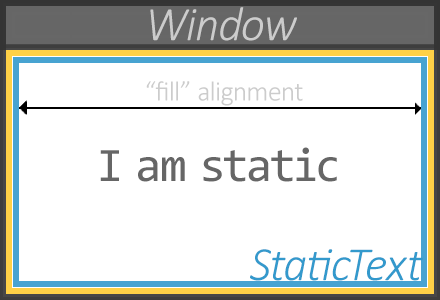1/ Text and Layout

Multiple PDF Exports Based on Layers

Given an InDesign document, wouldn't it be nice to automate the export of distinct PDFs based on custom sets of layers? For example, you need to make the layers “EN-1” and “EN-2” visible in the English version, while “FR-1”, “FR-2” and “EURO” must be seen in the French version, and so on.

The solution I suggest has two additional refinements: a forced layer is included in every export (cf the COMMON_LAYER_NAME constant), and any other layer having the same color is to be included as well.

Suggested code:

// ---
const LAYER_MAP =
{
//  pdfName:  [ layerName1, layerName2, etc ]
pdf1:  ["v1", "v2", "v4", "v5"],
pdf2:  ["v2", "v5"],
pdf3:  ["v1", "v3", "v5"],
};
const COMMON_LAYER_NAME = "Background";
const OUT_FOLDER = Folder.desktop;

const exportAllVersions = function(/*Document*/doc,  fd,LS,names,t,k,i,re)
//----------------------------------
{
// Presets.
// ---
const TOG =
[
{ printable:false, visible:false },   // 0 <-> OFF
{ printable:true,  visible:true  },   // 1 <->  ON
];
const PDF = +ExportFormat.pdfType;

// Checkpoints.
// ---
fd = Folder(OUT_FOLDER);
if( !fd.exists )
{ alert("The output folder does not exist."); return; }
// ---
if( (!doc) || Document!==doc.constructor)
{ alert("No document."); return; }
LS = doc.layers;
// ---
t = LS.itemByName(COMMON_LAYER_NAME);
if( !t.isValid )
{ alert("No `"+COMMON_LAYER_NAME+"` layer found."); return; }

// Deactivate all and coerce into arrays.
// ---
(LS=LS.everyItem()).properties = TOG;
names = LS.name;
LS = LS.getElements();

// Activate common layer(s) only. Color lookup.
// ---
k = callee.colorValue(t);
for( i=LS.length ; i-- ; )
{
k == callee.colorValue(t=LS[i])
&& ( t.properties=TOG, LS.splice(i,1), names.splice(i,1) );
}

// Generate separate PDF versions based on LAYER_MAP.
// ---
for( k in LAYER_MAP )
{
if( !LAYER_MAP.hasOwnProperty(k) ) continue;

re = RegExp( '^(?:' + LAYER_MAP[k].join('|') + ')\$' );
for(
i=names.length ;
i-- ;
LS[i].properties = TOG[+re.test(names[i])]
);

ff = File(fd + '/' + k + '.pdf');
doc.exportFile(PDF, ff);
}
}

exportAllVersions.colorValue = function(/*Layer*/ly,  r)
//----------------------------------
{
// UIColorsEnum | [R,G,B]
r = ly.getElements().layerColor;

return ( r instanceof Array ) ?
((r<<16)|(r<<8)|r) :
+r;
};

// Go!
// ---
exportAllVersions(app.properties.activeDocument);

• Original discussion: forums.adobe.com/message/11105640#11105640

Accessing the Parent Story of Whatever

Kals wrote: “In the script I'm working on, I need to get the parent story of the selection. This will typically be a Text object which is super easy with mySelection.parentStory. But in many cases it could be an object that doesn't have a parentStory property, like a table, row, column or cell. If it's a table, I worked out that I can get the parent story with mySelection.storyOffset.parentStory, but this doesn't work for rows, columns or cells (which have no storyOffset method). I could try navigating up the DOM tree with various checks and balances (…) but that seems like a crazy way to do something that I'd have thought would be simple.”

The discussion brought various tricks and snippets that perfectly do the job. The routine below is just an alternate approach based on DOM specifiers:

function getStory(/*?any*/x)
//----------------------------------
{
x||((x=app.properties.selection)&&(x=x));
if( x!==Object(x) || !('toSpecifier' in x) ) return false;

// Text objects already have a parentStory!
// ---
if( 'parentStory' in x ) return x.parentStory;

x = x.toSpecifier()
.match(/\/document\[.+?\/\/text-frame\[@id=\d+\]/);

if( !x || !(x=resolve(x)).isValid ) return false;
return x.parentStory;
};

• Original discussion: forums.adobe.com/message/11007574#11007574

Comparing Paragraph Spacing between Two Documents

How to check whether two versions of a document have the same “paragraph spacing” structure? Here are the rules of this curious game, as set by manuelb:

1. Sometimes the paragraph spacing in doc0 is a space after, while it is a space before in doc1, but it doesn't matter. The important is the space, not whether it is before or after.

2. The amount of space doesn't matter (as long as it is greater than zero.)

So, the user just wants to know if doc0 and doc1 match according to the above rules. As this is a yes-no problem, I've tried to answer the question with time performance in mind:

function compareParagraphSpacing(/*Document*/doc0,/*Document*/doc1,  a,i,n,t,j,bf0,af0,bf1,af1)
//----------------------------------
// Assuming than `doc1` and `doc2` share the *same story
// structure*, this function tells whether they also share the
// same basic 'spacing structure' between paragraphs (disregarding
// particular spacing values.) That is, considering any successive
// indices `i` and `i+1` and their corresponding paragraphs P_i,
// P_i+1 (in doc0) and Q_i, Q_i+1 (in doc1), the comparison
// succeeds under the following assertions:
// (a) If there is any space between P_i and P_i+1 --due to either
//     P_i.spaceAfter > 0 or P_i+1.spaceBefore > 0 (or both)-- then
//     that statement must hold for (Q_i, Q_i+1) as well.
// (b) If there is no space between P_i and P_i+1
//     --i.e P_i.spaceAfter = 0 and P_i+1.spaceBefore = 0-- then
//     the same must hold for (Q_i, Q_i+1).
// [REM] If one of the two documents has less paragraphs than the
// other, the comparison is performed on common paragraph indices
// using the lowest number.
{
const SEP = '§';
const R_SEP = RegExp(SEP,'g');
const R_ONE = RegExp(localize("%1([^0]|0[^%1])[^%1]*",SEP),'g');

if( !(doc0||0).isValid || !(doc1||0).isValid )
{ alert("Invalid documents."); return; }

// Space before and space after arrays.
// ---
for( a=[doc0,doc1], i=a.length, n=1/0 ; i-- ; )
{
t = a[i]=a[i].stories.everyItem().paragraphs.everyItem();
if( !(t||0).isValid )
{ alert("Invalid paragraphs."); return; }
t = a[i] = [t.spaceBefore,t.spaceAfter];
(t=t.length) < n && (n=t);
}

// Convert each space array into a trace string :: <1|0>+
// E.g  "01101" in `spaceBefore` trace means:
//      0_PAR_? 1_PAR_? 1_PAR_? 0_PAR_? 1_PAR_? . . .
// E.g  "11001" in `spaceAfter` trace means:
//      ?_PAR_1 ?_PAR_1 ?_PAR_0 ?_PAR_0 ?_PAR_1 . . .
//
// ---
for( i=a.length ; i-- ; )
{
n < (t=a[i]).length && (t.length=t.length=n);
for( j=2 ; j-- ;
t[j]=(SEP+t[j].join(SEP)).replace(R_ONE,SEP+'1').replace(R_SEP,'')
);

// Add dummy zeroes to allow in-sync merging.
// ---
t = t+'0'; // dummy trailing zero (before string.)
t = '0'+t; // dummy leading zero (after string.)
}

// Merge and compare through a 32-bit buffer.
// ---
bf0=a; af0=a;
bf1=a; af1=a;
for( t=i=0 ; (!t) && i < n ; i+=32 )
{
// Merge before/after for each stream, then XOR.
// ---
t = (parseInt(bf0.substr(i,32),2)
|parseInt(af0.substr(i,32),2))
^ (parseInt(bf1.substr(i,32),2)
|parseInt(af1.substr(i,32),2));
}

if( !t )
{
alert("Paragraph spacing structure of the 2 documents match :-)");
return;
}

// t != 0 indicates a mismatch at some point.
// ---
t = Math.min(32,n-i)-(t>>>0).toString(2).length;
i = 1+i+t;

alert( "Spacing mismatch at paragraph #" + i + " :-(" );
}

// TEST (Assuming TWO similar documents are available.)
//========================================
var docs = app.documents;
compareParagraphSpacing(docs,docs);

• Original discussion: forums.adobe.com/message/10450889#10450889

2/ Graphics and Geometry

Anchored Object Coordinates in CS6/CC

Andrii Chumak found an issue in resolving the coordinates of an anchored object using myAnchoredObj.resolve(...). The command works as expected in CS4, so the bug probably comes from CS6's changes in coordinate management (or maybe CS5.)

It is well-known that an anchored object (AO) belongs to a Story, which is not a geometric object. When an AO is visible (that is, when the associated anchor character is not in an overset region), its natural parent is the particular TextFrame that owns the anchor character. But this is not the actual parent of the AO in terms of coordinate spaces.

Unlike nested objects (“pasted into”) which have a true parent space, AOs need a kind of bridge to fit into the hierarchy of coordinate spaces. Unfortunately, this patch fails to connect AOs to regular Spread coordinate spaces in InDesign CS6 and later. The code

myAnchoredObj.resolve(
AnchorPoint.BOTTOM_LEFT_ANCHOR,
)

typically returns [0,0] whatever the location of the AO in the layout. So it seems that a fake coordinate space is created, based on the bottom-left corner of the AO bounding box. (Just my interpretation of what we can see, not a documented assertion.)

Of course you still have the option to read myAnchoredObj.geometricBounds and make cautious calculations from those data, noting that resolve(...) is powerful enough to interpret ruler coordinates.

I would suggest a different workaround, based on a strange but promising discovery. Apparently, AOs are associated to a more reliable structure at the PARENT_COORDINATES level. I don't think that's a true coordinate space, but since its origin coincides with the top-left corner of the TextFrame container (for whatever reason!) we should be able to work from there.

Note. — Contrary to what one might think, the so-called “parent space” of the AO is not the canonical inner space of the TextFrame. There are two noticeable hints about regular TextFrame spaces:
• Unlike usual PageItem spaces, their origin is initialized at the center location of the bounding box: myTextFrame.resolve(AnchorPoint.CENTER_ANCHOR, CoordinateSpaces.INNER_COORDINATES) returns [0,0] as long as the geometry of the frame is not altered.
• Also, the space origin follows the movement of the text frame, that is, its coordinates relative to the pasteboard evolves in a way that preserves the inner coordinates of anchor points.

Anyway, the query

myAnchoredObj.resolve(
<anyAnchorPoint>,
CoordinateSpaces.PARENT_COORDINATES
)

reveals the [x,y] coordinates of anyAnchorPoint in a coordinate system whose origin is the top-left corner of the TextFrame (not its center point). This coordinate system also seems to match the transform state of the TextFrame. Thus, what is left to us is to recover the TextFrame object from the AO and take advantage of transformation matrices to draw a coordinate path up to the Spread origin. (Click the below image to show the animation.)Schematically, we have the following information:

1. [x,y] in the 'fake space',
2. The origin of the fake space (i.e top-left anchor) in the TextFrame space,
3. The matrix that maps the TextFrame space to the Spread space.

From this we derive the coordinates of anyAnchorPoint relative to the Spread coordinate space.

Note that 3 is not necessarily a simple translation: the parent text frame may undergo complex transformations relative to the spread.

Suggested code:

//----------------------------------
// Given an anchored object `obj`, return the [x,y] coords
// of its center point in spread coordinate space. This func
// fixes the malfunction of `obj.resolve(<anchor>,<spreadCS>)`
// in CS6/CC.
{
const CS=CoordinateSpaces, AP=AnchorPoint;

// Get the parent TextFrame of obj.
// ---
txf = obj.parent.parentTextFrames;

// Coords of the center anchor in txf's 'TopLeftCorner'
// system (TLC) -- U can specify another ANCHOR if needed!
// ---
xy = obj.resolve(AP.CENTER_ANCHOR,CS.PARENT_COORDINATES);

// Coords of TLC's origin in the actual TextFrame space (TXF)
// --> `t` reflects the translation TLC->TXF.
// ---
t = txf.resolve(AP.TOP_LEFT_ANCHOR,CS.INNER_COORDINATES);

// TLC->SPD  :=  TLC->TXF × TXF->SPD
// ---
mx = app.transformationMatrices
.catenateMatrix(txf.transformValuesOf(
));

// Apply mx to xy.
// ---
return mx.changeCoordinates(xy);
}

// TEST. (Assuming an AO is selected.)
//========================================

Update 20-July-2019. — By digging deeper into this topic I discovered a better approach. Although you cannot trust myAO.resolve(…) for usual coordinate spaces above the inner space, it turns out that myAO.transformValuesOf(…) still works as expected in CS6-CC. So we can recover the transformation matrix that maps the inner space to the (true) spread coordinate space. This results in a much more elegant snippet:

//----------------------------------
//  New version based on the fact that -- unlike
// `obj.resolve` -- `obj.transformValuesOf` is reliable!
// ---
// Given an anchored object `obj`, return the [x,y] coords of its
// center point in spread coordinate space. This function corrects
// the malfunction of `obj.resolve(<anchor>,<spreadCS>)` in CS6/CC.
{
const CS=CoordinateSpaces, AP=AnchorPoint;

// Coords of the center anchor in AO's INNER SPACE
// (You can specify another ANCHOR if needed ;-)
// ---
xy = obj.resolve( AP.CENTER_ANCHOR, CS.INNER_COORDINATES );

// INNER-to-SPREAD matrix. In the context of `transformValuesOf()`,
// CS.SPREAD_COORDINATES refers to the actual spread coord. space
// (which would fail in the context of the `resolve()` method.)
// ---
mx = obj.transformValuesOf( CS.SPREAD_COORDINATES );

// Simply apply mx to xy :-)
// ---
return mx.changeCoordinates(xy);
}

This new version has another advantage: it still works with nested items within the anchored object (e.g. when the anchored object is a Group and your target object is an element of that group.)

• Original discussion: forums.adobe.com/message/10897624#10897624

Nice Function for Computing Polygon Areas

tmmls asked: “Does anyone know if it's possible to calculate the total coverage of an irregular polygon shape in Adobe Indesign?”

Our answer is based on Bogusław Jackowski's 2011 paper which exposes a generic method for computing the area enclosed by a Bézier spline. It's worth noting that the result is signed, meaning that auto-intersecting splines have positive and negative areas that may cancel each other out, as illustrated below.Suggested code:

var pathArea = function(/*[x,y][]*/ep,  r,n,i,P,Q,xa,ya,xb,yb,xc,yc,xd,yd)
//----------------------------------
// Based on gust.org.pl/bachotex/2011-en/presentations/JackowskiB_3c_2011
// Return AREA x 20 (signed result.)
{
for( r=0, n=ep.length, i=-1 ; ++i < n ; )
{
3 == (P=ep[i]).length ?
( xa=P, ya=P, xb=P, yb=P ) :
( xa=xb=P, ya=yb=P);

3 == (Q=ep[(1+i)%n]).length ?
( xc=Q, yc=Q, xd=Q, yd=Q ) :
( xc=xd=Q, yc=yd=Q);

r += (xb-xa)*(10*ya + 6*yb + 3*yc +    yd)
+  (xc-xb)*( 4*ya + 6*yb + 6*yc +  4*yd)
+  (xd-xc)*(   ya + 3*yb + 6*yc + 10*yd);
}
return r;
};

var splineArea = function(/*SplineItem*/o,  a,r,i,n,t)
//----------------------------------
// Visit every closed path and calculate the total area.
{
const CLOSED = +PathType.CLOSED_PATH;

a = o.paths.everyItem().getElements();
for(
r=0, n=a.length, i=-1 ;
++i < n && (t=a[i]).pathType==CLOSED ;
r+=pathArea(t.entirePath)
);

if( i < n ) throw "All paths must be closed.";

return (r/=20), 0 < r ? r : -r;
};

var getArea = function(/*obj|obj[]*/a,t,mu,i,r)
//----------------------------------
// Main function. Return the area in the form "<value> <unit>²".
// This function does not address splines that auto-intersect.
{
callee.Q||(callee.Q={
Q:               'q',
U:               'u',
POINTS:          'pt',
PIXELS:          'px',
PICAS:           'p',
MILS:            'mils',
MILLIMETERS:     'mm',
INCHES_DECIMEL:  'in',
INCHES:          'in',
HA:              'ha',
CICEROS:         'c',
CENTIMETERS:     'cm',
BAI:             'bai',
AMERICAN_POINTS: 'ap',
AGATES:          'ag',
});

(a instanceof Array) || (a=[a]);

// Set a consistent measurement unit.
// ---
while( 1 )
{
if( !(t=a) || 'function' != typeof t.toSpecifier )
throw "Invalid input."
t = t.toSpecifier().match(/^\/document\[@id=\d+\]/);
if( !t || !(t=resolve(t)) || !(t instanceof Document) )
throw "Invalid input.";
t = t.viewPreferences.horizontalMeasurementUnits;
app.scriptPreferences.measurementUnit = t;
mu = callee.Q[t.toString()]||'';
mu && (mu = ' ' + mu + '\xB2');
break;
}

for(
r=0, i=a.length ;
i-- ;
(o=a[i])&&o.hasOwnProperty('paths')&&(r+=splineArea(o))
);

return String(r) + mu;
};

// TEST. (Assuming something is selected.)
//========================================
var sel = app.selection;

Smart-Rescaling Images within a Text Flow

Aman Mittal wrote: “I am working on a document with 500 images and all of them run beyond the text frame size (…) Hence, to fit them to the frame, I tried to use Transform/Scale and set the percentage value to less than 100% and the image got fit into the text frame. However, the problem is with a single selection. Selecting an image every time and setting the scale for 500 objects is a time consuming task. I tried using Object style but it does not incorporate Scale option in its palette…”

Due to additional constraints, this task is not as easy to address as originally supposed. Basically, the goal is to rescale inline images in a flow of threaded text frames, assuming Smart Text Reflow is turned on. Rescaling needs to be done proportionnally, with respect to the dimensions of the frame which inline objects belong to. But as we are facing overset text issues, how could we tell which text frame an inline object would belong to while such container remains unspecified? Also, objects have no visible bounding box as long as they cannot be laid out, which makes impossible to compute their dimensions.

Key idea: dramatically downscale inlines first, in order to reveal every imageable object in the flow, then compute width/height ratio relative to the container and perform final adjustments. Many other points have been clarified in the original discussion. The below code is one solution among others. This is a perfect example of a fine-tuneable script that already automates a pretty complicate work.

function fixAndDownscaleInlines(  q,doc,t,wso,n,items,chars,z,c,o,aos,k,wh,xy,x,y)
//----------------------------------
// This function proportionnaly downscale every *inline* or
// *aboveLine* anchored item found in the document, so that:
// 1. Target width does not exceed parent frame width (PFW.)
// 2. Targets whose width is *under* PFW are not touched.
// 3. Objects whose height exceeds the parent frame height (then
//    causing overset issues that SmartTextReflow can't fix) are
//    temporarily downscaled so that the algorithm can eat them.
//    When available back, those objects are adjusted to the frame
//    height unless width adjustment is required instead (to
//    prevent them from violating rule 1.)
// [REM] Smart Text Reflow must be turned on and properly set.
// [REM] Force inline anchoring when aboveLine is found.
// [REM] Use `document.recompose()` to help smartReflow update.
{
// Cache and constants.
// ---
q = (callee.Q||callee.Q={});
const EPSILON = 1e-3,
DOWN = 100, // Downscaling factor
ANCH = +AnchorPosition.ANCHORED,
ABOV = +AnchorPosition.ABOVE_LINE,
INLN = +AnchorPosition.INLINE_POSITION,
// ---
CPAR = +CoordinateSpaces.parentCoordinates,
CINN = +CoordinateSpaces.innerCoordinates,
BVSB = +BoundingBoxLimits.outerStrokeBounds,
// ---
PAR0 = [+AnchorPoint.topLeftAnchor, BVSB],
PAR1 = [+AnchorPoint.bottomRightAnchor, BVSB],
INN0 = PAR0.concat(CPAR),
INN1 = PAR1.concat(CPAR),
MATX = [1,0,0,1, 0,0];

// Checkpoint.
// ---
if( !(doc=app.properties.activeDocument) )
{ alert("No document!"); return; }
if( !(t=doc.textPreferences).properties.smartTextReflow )
{ alert("SmartTextReflow must be turned on!"); return; }
if( !(t=doc.pages.appliedMaster) )
{
if( !confirm("No applied master on first page. Fix this?") ) return;
t = doc.pages.appliedMaster = doc.masterSpreads;
}
if( !t.primaryTextFrame )
{ alert("No primary frame on spread "+t.name+"."); return; }

// [ADD180621] Prepare text reflow.
// ---
doc.pages.itemByRange(1,-1).remove();
t.properties = {
deleteEmptyPages:          true,
limitToMasterTextFrames:   true,
};

// Get anchored items from all stories,
// and respective parent char.
// ---
t = doc.stories.everyItem().texts.everyItem().pageItems;
if( !t.length ){ alert("No anchored item."); return; }
items = (t=t.everyItem()).getElements(); // anchored items
chars = t.parent;                        // anchor characters

// Safe scaling options are definitely needed.
// ---
wso = +app.transformPreferences.whenScaling;
wso==t ? (wso=0) : (app.transformPreferences.whenScaling=t);

//  Patch. Looping here from top to bottom (in
// each story stream) we try to restore missing bounding boxes
// due to 'formal heights' being higher than the parent frame.
// A flag is then set in q[_id] to save those special cases.
// ---
for( n=items.length, z=-1 ; ++z < n ; )
{
// `o` is a PageItem which typically contains
// a Graphic which typically controls a Link.
// ---
o = items[z];
if( o.properties.visibleBounds ) continue;

// `o` has no parent text frame because it's too high!
// Let's temporarily apply a hard downscaling to it.
// ---
if( !(x=o.properties.horizontalScale) ) continue;
if( !(y=o.properties.verticalScale) ) continue;
o.horizontalScale = x/DOWN; // 1%
o.verticalScale = y/DOWN;   // 1%
q['_'+o.id] = 1; // flag
}

//  Force recomposition now.
// ---
doc.recompose();

// Scan each anchor character and its respective parent frame
// (if any!)
// ---
for( z=0 ; (c=chars.pop()) && (o=items.pop()) ; )
{
// c in overset text -> no parent available.
// ---
if( !(t=(c.parentTextFrames||0)) ) continue;

// Filtering conditions on `o` (you may add your own.)
// Here we check that the anchor is inline/aboveLine.
// ---
aos = o.properties.anchoredObjectSettings||0;
if( (!aos) || ANCH==+aos.anchoredPosition ) continue;

// [FIX180621] Force inline.
// ---
aos.anchoredPosition = INLN;

// Visible width and height of the text frame
// (if not in cache yet.)
// ---
if( !(wh=q[k=t.id]) )
{
wh = q[k] = t.resolve(PAR1,CINN);
xy = t.resolve(PAR0,CINN);
wh -= xy;
wh -= xy;
}

// Temporarily store the (w,h) of `o` (in parent space.)
// ---
t = o.resolve(INN1,CPAR)
.concat(o.resolve(INN0,CPAR));
t -= t;
t -= t;
t.length = 2;

// The x factor is required in either case.
// ---
x = wh/t;

// If `o` has been previously downscaled to make it show
// up, we need to find the *smallest fit factor*.
// ---
y = q['_'+o.id] ? wh/t : false;

// If `o` hasn't been downscaled, prevent it from being
// upscaled. ("Width shorter than the frame width -> do
// nothing.")
// ---
if( (!y) && 1 <= x+EPSILON ) continue;

// Rescale.
// ---
y && y < x && (x=y);
MATX = MATX = x;
o.transform(CPAR,INN0,MATX);
++z;
}

wso && (app.transformPreferences.whenScaling=wso);
alert( "Processed items: " + z);
};

app.doScript(fixAndDownscaleInlines, void 0, void 0,
UndoModes.entireScript, "FixAndDownscaleInlines");

• Original discussion: forums.adobe.com/message/10450182#10450182

3/ ScriptUI Stuff

Positioning a DropDown Next to a Panel Title

There is no direct way to position a ScriptUI element in the region of a panel title. To get this result,one option is to create a stack —that is, a group with orientation set to "stack"— and to superimpose the components.

var w = new Window('dialog', "My Dialog");

var stack = w.add('group');
stack.orientation = 'stack';

var pageSizePanel = stack.add
('panel', void 0, 'Page Size', {borderStyle:'gray'});

pageSizePanel.minimumSize = [300,200];
pageSizePanel.margins = [10,30,10,10];
pageSizePanel.alignChildren = ['left','top'];

// etc.

dropdown.selection = 1;
dropdown.parent.alignment = ['left','top'];
dropdown.parent.margins = [100,0,0,0]; // Allows to left-indent the dropdown as desired

w.show();

• Original discussion: forums.adobe.com/message/10980148#10980148

How to Center a StaticText

Given a ScriptUI Group G containing a StaticText S, you may suppose that having both G and S alignment property set to "center" would maintain the text UI-centered when changing. This is not the case, because G and S area are fixed at layout time by the AutoLayoutManager,and this won't update properly if the text length changes.

The usual solution is to make your components "fill"-aligned and set to "center" the justify property of the StaticText,

// ...

G.alignment = S.alignment = "fill";
S.justify = "center"

// ...

which then allocates enough room to the text area:• Original discussion: forums.adobe.com/message/10945955#10944950

Preparing ScriptUIImage Instances

There are many ways to declare and manage responsive images in a ScriptUI interface. My preferred is based on sprites, but we can deal with separate ScriptUIImage instances as well. The question is, When do we need to create those instances? Isn't it a good option to have them already available when the UI launches? This, indeed, should reduce memory consumption and refreshing time.

You can invoke the ScriptUI.newImage() method on a stringified PNG. It then returns a pure ScriptUIImage object that you can store, for example, in an associative array for later use. The below code illustrates that approach in a full example. Look how the ANCHORS set is prepared even before declaring the dialog:

const KEYS = function(/*obj*/o,  a,k)
//----------------------------------
{
a=[];
for( k in o )
o.hasOwnProperty(k)&&(a.push(k),a[k]=o[k]);
return a;
};

const IM = function(/*str*/png)
//----------------------------------
{
return ScriptUI.newImage(png);
};

Image.prototype.refresh = Function('wh',
//----------------------------------
[
"wh=this.size;",
9 <= parseFloat(app.version) ?
"this.size=[1+wh,1+wh];" :
"",
"this.size = [wh,wh];"
].join(''));

// Anchors (pngs + names.)
// ---
const ANCHORS = KEYS({
'None':          IM("\x89PNG\r\n\x1A\n\0\0\0\rIHDR\0\0\0'\0\0\0'\b\x06\0\0\0\x8C\xA3Q5\0\0\0\tpHYs\0\0\v\x12\0\0\v\x12\x01\xD2\xDD~\xFC\0\0\x01<IDATX\xC3c```\xF8\x8F\r\x9B\x9A\x9A\x9E\xF9\xFF\xFF?\x03>\f\x04\x0E\xB8\xF4GGG\xCF\xA0D?\x107\x80\x14\xFCG\x077o\xDE\xFCoggw\x81\x18\xC3\xD3\xD3\xD3\xDF\xA3\xEB_\xBBv\xED\xFF\x8C\x8C\x8C\x05\xC4\xE8/--\xFD\x8FM?^\xC7Y[[\xDF\x81\xFA\f\x1F.\0:\xEE36\xC3\x13\x13\x13w\x10\xA3\x9F,\xC7\x99\x99\x99\xFD\xC7\x13\xE4p\x9C\x92\x92\x82\xD5\xF0\x80\x80\0\xA2\xF4\x0F\xED\x90\x039\x06\x19\xEF\xDC\xB9\x93\xE84\x17\x1E\x1E\xFE\x19]\xFF\xE4\xC9\x93\x89Ns\xA0\x90\xC7\xA6\x1F\xEC8P\xAE\x049\xC4\xCA\xCA\xEA\x05(*Al\x10\xD6\xD7\xD7\x9FN\x84\xE1\x060\xFD\xE8\xD8\xD5\xD55\x8B\x18\xFD0\xF5\xE8\xF6\x03\xE5\x12\x90\x156@\xA3\x98a 06\xFBG\x1D7\xEA\xB8Q\xC7\x8D:n\xD8;\x0EW\x85\xAC\xA5\xA5u\x82\b\x03q\xB6\xC7\xBC\xBD\xBD'P\xA2\x7F\xE8\xB6\xE7F\x1D7\xE4\x1D7\xDA\x9E\xC3\xA1\x7F\xB4=7\xEA\xB8Q\xC7\x8D:n\xD4q\x83\xD5q\xA3\xED9<\xFA\xC9j\x95\x80\xEA:\xA8\x02|xAZZ\xDAwl\x86GEE] F\xFF\xF0\x1B\x9F\x1B4\xD1:\xE8\xDBs\xE8\x98\xDE\xED9t\fj\xCF\x01\0\x8F\x8F>\f5c\xDC\xF0\0\0\0\0IEND\xAEB`\x82"),
'Bottom Left':   IM("\x89PNG\r\n\x1A\n\0\0\0\rIHDR\0\0\0'\0\0\0'\b\x06\0\0\0\x8C\xA3Q5\0\0\0\tpHYs\0\0\v\x12\0\0\v\x12\x01\xD2\xDD~\xFC\0\0\x01:IDATX\xC3c```\xF8\x8F\rkii\x9D\xF8\xFF\xFF?\x03>\f\x04\x0E\xB8\xF4{{{O\xA0D?\x107\x80\x14\xFCG\x077o\xDE\xFCoggw\x81\x18\xC3\xD3\xD3\xD3\xDF\xA3\xEB_\xBBv\xED\xFF\x8C\x8C\x8C\x05\xC4\xE8/--\xFD\x8FM?^\xC7Y[[\xDF\x81\xFA\f\x1F.\0:\xEE36\xC3\x13\x13\x13w\x10\xA3\x9F,\xC7\x99\x99\x99\xFD\xC7\x13\xE4p\x9C\x92\x92\x82\xD5\xF0\x80\x80\0\xA2\xF4\x0F\xED\x90\x039\x06\x19\xEF\xDC\xB9\x93\xE84\x17\x1E\x1E\xFE\x19]\xFF\xE4\xC9\x93\x89Ns\xA0\x90\xC7\xA6\x1F\xEC8SS\xD33 \x87XYY\xBD\0E%\x88\r\xC2\xFA\xFA\xFA\xD3\x890\xDC\0\xA6\x1F\x1D\xBB\xBA\xBAf\x11\xA3\x1F\xA6\x1E\xDD~\xA0\\\x02\xB2\xC2\x06h\x143\f\x04\xC6f\xFF\xA8\xE3F\x1D7\xEA\xB8Q\xC7\r{\xC7\x8D\xB6\xE7\xF0\xE8'\xABU2\xEA\xB8!\xEF\xB8\xD1\xF6\x1C\x0E\xFD\xA3\xED\xB9Q\xC7\x8D:n\xD4q\xA3\x8E\x1B\xAC\x8E\xC3\xD3\x9E\x1A\x1C\xED9\x1CxpT\xFC8\xF0\v\xA8\x02|xAZZ\xDAwl\x86GEE] F?\xB9\x8E\x1B\x1C\xE3sC1Z\x07\xBE=\x07\x04g@\x0E\xC1\x82\xE9\xDA\x9EC\xC7\xA0\xF6\x1C\0\x9B\x11\xEE\x83W>)\xAD\0\0\0\0IEND\xAEB`\x82"),
'Bottom Right':  IM("\x89PNG\r\n\x1A\n\0\0\0\rIHDR\0\0\0'\0\0\0'\b\x06\0\0\0\x8C\xA3Q5\0\0\0\tpHYs\0\0\v\x12\0\0\v\x12\x01\xD2\xDD~\xFC\0\0\x014IDATX\xC3c```\xF8\x8F\rkii\x9D\xF8\xFF\xFF?\x03>\f\x04\x0E\xB8\xF4{{{O\xA0D?\x107\x80\x14\xFCG\x077o\xDE\xFCoggw\x81\x18\xC3\xD3\xD3\xD3\xDF\xA3\xEB_\xBBv\xED\xFF\x8C\x8C\x8C\x05\xC4\xE8/--\xFD\x8FM?^\xC7Y[[\xDF\x81\xFA\f\x1F.\0:\xEE36\xC3\x13\x13\x13w\x10\xA3\x9F,\xC7\x99\x99\x99\xFD\xC7\x13\xE4p\x9C\x92\x92\x82\xD5\xF0\x80\x80\0\xA2\xF4\x0F\xED\x90\x039\x06\x19\xEF\xDC\xB9\x93\xE84\x17\x1E\x1E\xFE\x19]\xFF\xE4\xC9\x93\x89Ns\xA0\x90\xC7\xA6\x1F\xEC8SS\xD33 \x87XYY\xBD\0E%\x88\r\xC2\xFA\xFA\xFA\xD3\x890\xDC\0\xA6\x1F\x1D\xBB\xBA\xBAf\x11\xA3\x1F\xA6\x1E\xDD~\xA0\\\x02\xB2\xC2\x06h\x143\f\x04\xC6f\xFF\xA8\xE3F\x1D7\xEA\xB8Q\xC7\r{\xC7\x8D\xB6\xE7\xF0\xE8'\xABU2\xEA\xB8!\xEF\xB8\xD1\xF6\x1C\x0E\xFD\xA3\xED\xB9Q\xC7\x8D:n\xD4q\xA3\x8E\x1B\xAC\x8E\x1Bm\xCFQ\xDBq\xA0\xBA\x0E\xAA\0\x1F^\x90\x96\x96\xF6\x1D\x9B\xE3\xA2\xA2\xA2.\x10\xA3\x9F,\xC7\xD1k|nhG\xEB@\xB6\xE7\xF0:\x0EW{\x8C^\xED9 \xBE\x80\x03'\0\0\xFF(\xEE\xCB;\xDE\x8C\x11\0\0\0\0IEND\xAEB`\x82"),
'Center':        IM("\x89PNG\r\n\x1A\n\0\0\0\rIHDR\0\0\0'\0\0\0'\b\x06\0\0\0\x8C\xA3Q5\0\0\0\tpHYs\0\0\v\x12\0\0\v\x12\x01\xD2\xDD~\xFC\0\0\x01EIDATX\xC3\xED\x98\xB1\x8A\x83@\x10\x86\xF7ylL\xA3\x85\x82\xA4\xB1\xB2\xB1\x13\xACN\x10\x95\xAB\xD2\xD8\xE7\x11\x0E,\xAE\xF5\x05\xEC\xCF7\xB8\"\x85\xADp\x0Fp]\n\x8B\x94\x93\xDD\xC3\x84\xB0l\x96!\xE2%&#| \xB8\xFF\xEC\xE7\xAE\xE0\xB0\x8C1\x06*\f\xC3\xF8\x06\0\xA6\x83_\xEBk\xF9 \b>\xA6\xE49[1\0\xE4\xAB\xEF{\xF0<\xAF\xC3\x14\xCF\xF3|/\xE7\x9B\xA6\x81\xA2(jL\xBE,KP\xE5\xB5r\xAE\xEB\xFE\x8Co\xA6c\xC3\xE5\x06U\xF1\$I\xBE0\xF9\x9B\xE4l\xDB\x06\xCD\x92\x9FI\xD3TY<\fCT~\xD9+'d.i\xDB\x16\xFD\xCDEQ4\xC8\xF9\xAA\xAA\xD0\xDF\x9CXyU\xFEO\xCE\xB2\xAC\x9D\x10q\x1C\xE7Wl\xA5\xB8\x17\x98\xA6\xF9\x89(\xBE:\xE5e|\xDF\x7F\xC7\xE4O\xE3\xE5\xF9\xF9\xB3\xB7\xCB\x81\xDBq\x8B\xD9=P\xCDOr\$Gr\$\xF7\xF4rs\xF5s\x9C\xFB\xF7s\x9C\xFD\x95\xE25&\x7FSWBr\x8B\x97\x9B\xD2\xCFq\x86)r\xB3\xF6s\x9C\x1D\xA7S@\xFD\x1C\xC9\x91\x1C\xC9\x91\xDC\xA2\xE5\xE6\xEA\xE7^\xF7|N\xFC\xEB\xC6\x01:\xEA,\xCB\x0E\xAA\xE2q\x1Cw\x98\xFC\xF3\x9D\xCF=\xCC\xB6>\xFC\xF9\x9C\xCC\x7F\x9F\xCF\xC9\x88~\xEE\b\x82z\xE7\xEDR\xDD\xF9<\0\0\0\0IEND\xAEB`\x82"),
'Left':          IM("\x89PNG\r\n\x1A\n\0\0\0\rIHDR\0\0\0'\0\0\0'\b\x06\0\0\0\x8C\xA3Q5\0\0\0\tpHYs\0\0\v\x12\0\0\v\x12\x01\xD2\xDD~\xFC\0\0\x01AIDATX\xC3c```\xF8\x8F\rkii\x9D\xF8\xFF\xFF?\x03>\f\x04\x0E\xB8\xF4{{{O\xA0D?\x107\x80\x14\xFCG\x077o\xDE\xFCoggw\x81\x18\xC3\xD3\xD3\xD3\xDF\xA3\xEB_\xBBv\xED\xFF\x8C\x8C\x8C\x05\xC4\xE8/--\xFD\x8FM?^\xC7Y[[\xDF\x81\xFA\f\x1F.\0:\xEE36\xC3\x13\x13\x13w\x10\xA3\x9F,\xC7\x99\x99\x99\xFD\xC7\x13\xE4p\x9C\x92\x92\x82\xD5\xF0\x80\x80\0\xA2\xF4\x0F\xED\x90\x039\x06\x19\xEF\xDC\xB9\x93\xE84\x17\x1E\x1E\xFE\x19]\xFF\xE4\xC9\x93\x89Ns\xA0\x90\xC7\xA6\x1F\xEC8SS\xD33 \x87XYY\xBD\0E%\x88\r\xC2\xFA\xFA\xFA\xD3\x890\xDC\0\xA6\x1F\x1D\xBB\xBA\xBAf\x11\xA3\x1F\xA6\x1E\xDD~\xA0\\\x02\xB2\xC2\x06h\x143\f\x04\xC6f\xFF\xA8\xE3F\x1D7\xEA\xB8Q\xC7\r{\xC7\xE1\xA9\x94\x07G{\x0E\x07\x1E\x1C\xED\xB9Q\xC7\x8D:\x0E\xCDp\x9A\xB6\xE7\x80\xE0\f\xC8!@\xFC\x02\xE6((\x1Em\xCF\xE1\xB3\x7F\xD4q\xA3\x8E\x1Bu\xDC\xA8\xE3\x86\xBD\xE3pU_\xA3\xE3s\xE4\x8E\xCF\x81\xEA:\xA8\x02|xAZZ\xDAwl\x86GEE] F\xFF\xF0\x1B\x9F\x1B4\xD1:\xE8\xC7\xE7\xD01\xBD\xC7\xE7\xD01\xA8=\x07\0\xD9B\xEE\x83-\xBB>\x98\0\0\0\0IEND\xAEB`\x82"),
'Right':         IM("\x89PNG\r\n\x1A\n\0\0\0\rIHDR\0\0\0'\0\0\0'\b\x06\0\0\0\x8C\xA3Q5\0\0\0\tpHYs\0\0\v\x12\0\0\v\x12\x01\xD2\xDD~\xFC\0\0\x019IDATX\xC3c```\xF8\x8F\rkii\x9D\xF8\xFF\xFF?\x03>\f\x04\x0E\xB8\xF4{{{O\xA0D?\x107\x80\x14\xFCG\x077o\xDE\xFCoggw\x81\x18\xC3\xD3\xD3\xD3\xDF\xA3\xEB_\xBBv\xED\xFF\x8C\x8C\x8C\x05\xC4\xE8/--\xFD\x8FM?^\xC7Y[[\xDF\x81\xFA\f\x1F.\0:\xEE36\xC3\x13\x13\x13w\x10\xA3\x9F,\xC7\x99\x99\x99\xFD\xC7\x13\xE4p\x9C\x92\x92\x82\xD5\xF0\x80\x80\0\xA2\xF4\x0F\xED\x90\x039\x06\x19\xEF\xDC\xB9\x93\xE84\x17\x1E\x1E\xFE\x19]\xFF\xE4\xC9\x93\x89Ns\xA0\x90\xC7\xA6\x1F\xEC8SS\xD33 \x87XYY\xBD\0E%\x88\r\xC2\xFA\xFA\xFA\xD3\x890\xDC\0\xA6\x1F\x1D\xBB\xBA\xBAf\x11\xA3\x1F\xA6\x1E\xDD~\xA0\\\x02\xB2\xC2\x06h\x143\f\x04\xC6f\xFF\xA8\xE3F\x1D7\xEA\xB8Q\xC7\r{\xC7\x8D\xB6\xE7F\x1D7\xEA84\xC7\rd{\x0E\xAF\xE3\x06\xBA=\x07\xC4\x17\xA0\xF8\x05\xD4Q0\xFEh{n\xD4q\xA3\x8E\x1Bu\xDC\xA8\xE3\x06\xD4q\xA3\xED9<\xFA\xC9\x1Ae\x02\xD5\xB5P\x05\xF8\xF0\x82\xB4\xB4\xB4\xEF\xD8\f\x8F\x8A\x8A\xBA@\x8C\xFE\xE17>7h\xA2u\xD0\x8F\xCF\xA1cz\x8F\xCF\xA1cP{\x0E\0\xABh\xEE\xEF\xC9e\xF2\xDA\0\0\0\0IEND\xAEB`\x82"),
'Top Center':    IM("\x89PNG\r\n\x1A\n\0\0\0\rIHDR\0\0\0'\0\0\0'\b\x06\0\0\0\x8C\xA3Q5\0\0\0\tpHYs\0\0\v\x12\0\0\v\x12\x01\xD2\xDD~\xFC\0\0\x01FIDATX\xC3c```\xF8\x8F\rkii\x9D\xF8\xFF\xFF?\x03>\f\x04\x0E\xB8\xF4\x03\xF1\x04\n\xF57\x80\x14\xFCG\x077o\xDE\xFCoggw\x81H\xC3\xDF\xE30|\x011\xFAKKK1\xEC_\xBBv-~\xC7Y[[\xDF\x81Z\x8E\x0F\x17\0\xF1g\x1C\x8E\xDBA\x8C~\xB2\x1Cgff\x86+\xB8\xA9\x8A\x87v\xC8\x81\x1C\x83\x8Cw\xEE\xDCIJ\x9A\xC3\xE58\xA2\xD2\\JJ\n\x86\xFD\x93'O\x868\xCE\xD4\xD4\xF4\f\xC8!VVV/@Q\tb\x83\xB0\xBE\xBE\xFEt\"\f7\0\xE23@|\x01\v\xCE\"F?\xCC>t\xFB\x81r\t\xC8\n\x1B\xA0Q\xCC0\x10\x18\x9B\xFD\xA3\x8E\x1Bu\xDC\xA8\xE3F\x1D7\xEC\x1D\x87\xABB\xA6\xB4=\xE7\xED\xED=\xF0\xED\xB9\xF4\xF4\xF4\xF7\xE8\xFAA\x15wFF\xC6\x02b\xF4\x93\xD5*\x19u\xDC\x90w\x1C%\xED\xB9\xF0\xF0\xF0\xCF\xD8\xDAc\xC4:\x8E\xA6\xED9\x98~t\xEC\xEA\xEA:\xDA\x9E\x1Bu\xDC\xA8\xE3F\x1D7\xEA\xB8!\xED\xB8\xD1\xF6\x1C\x1E\xFDd\xB5J@u\x1DT\x01>\xBC --\xED;6\xC3\xA3\xA2\xA2.\x10\xA3\x9F\xA6\xE3s\xA0V\x056\xC3\x03\x02\x02h7>7h\xA2u\xD0\xB7\xE7\xD01\xBD\xDBs\xE8\x18\xD4\x9E\x03\0\xD6\x8F\xE8h\xF2\xC8\xECy\0\0\0\0IEND\xAEB`\x82"),
});

// Dialog.
// ---
var w = new Window('dialog', "Select the reference point");
( w.ancGroup = w.add('group') )
.spacing = 15;
( w.ancList  = w.ancGroup.add('dropdownlist',void 0,ANCHORS) )
.minimumSize = [200,24];
( w.ancImg   = w.ancGroup.add('image',void 0,ANCHORS.None) )
.imgName = ANCHORS[w.ancList.selection=0];
( w.btnGroup = w.add('group') )
.spacing = 15;
( w.ok = w.btnGroup.add('button',void 0,"OK") );
( w.ko = w.btnGroup.add('button',void 0,"Cancel") );

// Event managers.
// ---
w.ancImg.onDraw = function onDraw()
//----------------------------------
{
this.graphics.drawImage(ANCHORS[this.imgName],0,0);
};

w.ancList.onChange = function onChange(  s,t)
//----------------------------------
{
(s=this.selection)
&& ((t=this.window.ancImg).imgName=s.text)
&& t.refresh(); // trigger onDraw (indirectly.)
};

var result = w.show();

resulting in:• Original discussion: forums.adobe.com/message/10448268#10448268

GitHub page: github.com/indiscripts